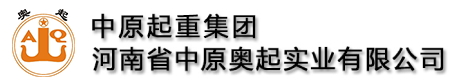全国统一服务热线:13782575673

# 欧式起重机小车的设计计算实例

### 来源：未知   发布时间：2021-03-05 10:07  点击量：

设计某小欧式起重机小车，其设计参数为：额定起重量Q=32t;工作级别A5;起升高度H=17m;起重机跨度S=22500mm;起升速度V起=0.32～3.2m/min;小车运行速度V小=2～20m/min。

(1)32t欧式起重机小车横梁设计计算

根据定滑轮在小车横梁里的结构布置，设计截面尺寸见图3-56。Q=320000N;a=900mm;I1=I4=3.96×108mm4;I2=I3=7.32×108mm4;t1=16mm;t2=12mm;t3=20mm;S1=S4=1.26×106mm3;S2=S3=1.73×106mm3;y2=y3=329mm;d=100mm;E=2.1×105N/mm2;L=2200mm强度符合要求。

根据小车横梁的结构设计截面尺寸(图3-56)，验算刚度。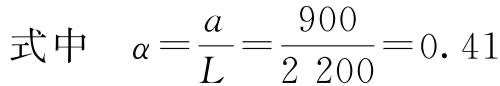刚度符合要求。

(2)32t欧式起重机小车端梁计算(图3-57)Q=320000N;Gx=20000N;a=180mm;b=980mm;c=417mm;I1=2.1×107mm4;I2=2.1×108mm4;I3=332029916mm4;I5=209242013mm4;I6=9069917mm4;y2=174mm;y3=222mm;y5=174mm;S1=188016mm3;S2=826640mm3;S3=1244864mm3;S5=826640mm3;S6=109856mm3小车端梁计算符合要求。

(3)小车运行机构设计计算

以下为小车“三合一”减速器选型计算。

①小车摩擦阻力计算②小车坡道阻力的计算

坡度角αsinα=0.001

坡道阻力Fp=(Q+G小)×sinα=(3.2×105+4.0×104)×0.001=360N

③小车运行静阻力的计算

Fj=Fm+Fp=4968+360=5328N

④小车运行静功率计算PN=kd·Pj=1.03×0.95=0.98kW

⑤小车运行静阻力矩的计算

MP1=Fj×R=5328×0.125=666N·m

式中　R——小车车轮的半径，R=D/2m。

小车满载直线运行产生的惯性力矩：加速度取0.05。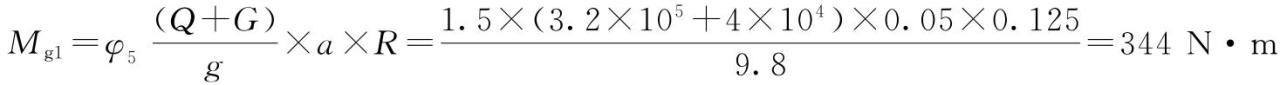MH=MP1+Mg1=666+344=1010N·m

小车为对边驱动型式，使用两个减速器驱动，1个齿轮箱应承载MH/2=505N·m。

Na=V/3.14×D=25.5

⑥减速器选型

推荐选取国内恒星科技品牌“三合一”F系列减速器：功率1.1kW;fb=2.0;i=55.42;na=25;Ma=410N·m。

Ma×fb=410×2.0=820N·m>MH/2=505N·m

所选减速器符合要求。

⑦小车最大轮压计算

小车最大轮压是R1和R6的大值，R6=94.5kN>R1=93.5kN。所以，Pmax=R6=94.5kN为小车的最大轮压(主动轮压)，R1=93.5kN为被动轮压，R1/R6=93.5/94.5=0.99，被动轮、主轮压比非常理想，被动轮压接近主动轮压但小于主动轮压，在实践中一般不会出现持久打滑现象。

⑧小车车轮设计计算小车轨道选方钢40mm×40mm，初选实心ф250mm直径车轮，车轮有效接触宽度l=40-6=34mm;

车轮材料选DG42CrMo取σb=1080N/mm2，许用轮压比k=8.5。

允许轮压：PL=kDlC=8.5×250×34×1.03×1=74.4kN

疲劳强度校核：Pmean=66.3kN≤PL=74.4kN，满足要求。

静强度校核：94.5kN=Pmax≤1.9kDl=1.9×8.5×250×35=141.3kN，满足要求。

(4)起升机构的设计计算

①钢丝绳的选型计算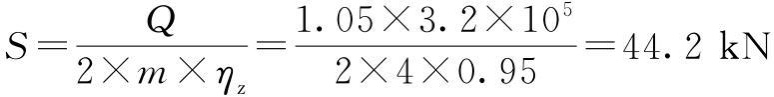所需钢丝绳最小破断拉力：S0=S×n=44.2×4.5=198.9kN

选择某国外钢丝绳，查样本型号为：15-8×WS(26)+IWRC-1960

样本上钢丝绳最小破断拉力为202.7kN，所选钢丝绳符合要求。

②起升电机的选型计算

起升机构总传动效率：η=η1×η2×η3=0.95×0.96×0.95=0.87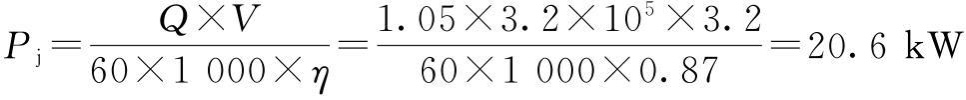选某国际品牌“三合一”变频起升电机，型号DFV200L6B(S1);n=970r/min;P=18.5kW。

电机实际额定功率Pe=1.3P=1.3×18.5=24.05kW>Pj=20.6kW，所选电机符合要求。

③起升减速器的选型计算

初步取卷筒名义直径尺寸：D=450mm

钢丝绳公称直径：d=15mm

卷筒计算直径：D0=d+D=15+450=465mm

起升动载系数：Φ2=1.12

减速器低速端所承受的扭矩：

T=Φ2×S×D0=1.12×4.42×104×0.465=23.02kN·m

卷筒转速：减速器速比：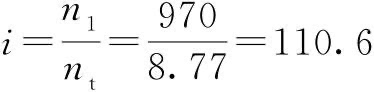确定齿轮箱的额定功率：P1N≥Pj·f1·f2=20.6×1.1×1=22.7kW

最大启动扭矩：TA=1.8×Mn=1.8×176.7=318.1N·m(查电机样本得Mn)

峰值扭矩校核：P1N=22.7kW≥TA·n1·f3/9550=318.1×970×0.7/9550=22.62kW

选某国际品牌减速器：(额定功率25.7kW)i=112输出扭矩25.7kN·m。

综上述计算结果可知，减速器的选择符合规范要求。

④制动器的选型计算起升“三合一”电机带制动单元，给厂家提额定供制动力矩230.2N·m。

⑤卷筒联轴器的选型计算

查工作级别系数K2=1.4(表3-6)卷筒支承轴承的效率ηT=0.98

卷筒钢丝绳总拉力：∑S=(Q+Q0)/m×η=(1.05×3.2×105)/4×0.98=85.7kN

联接处所受实际转矩：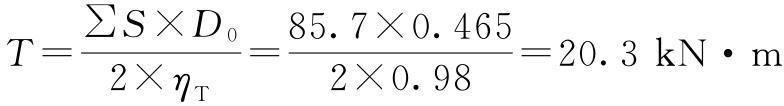联接处的计算转矩：TK=T×K2×Φ6=20.3×1.4×1.06=30.1kN·m

联接处实际承受的径向载荷：选某国内品牌DC联轴器：DC035A，Tmax=45kN·m，Fmax=50kN

Fr=43.1kN≤Fmax+(Tmax-TK)×K1=50+(45-30.1)×3.7=105.13kN

符合设计要求

⑥卷筒设计计算(卷筒光杆Lg长度部分计算)

在设计小车时得以下数据：b=20mm;c1=46mm;δ=148mm;H3=565mm;Dd=314mm;Dg=314mm;Dw=400mm;Hf=305mm。Hf符合要求，钢丝绳在小车横梁底部不会发生干涉。

Dw-Dd-d≥Lg≥Dw-Dd-d-2H0·tan3.5°

400-314-15≥Lg≥400-314-15-2×840×tan3.5°

即71≥Lg≥-37mm，负值说明，只要Lg小于71就可以。

取Lg=50mm

主卷筒选择P=17mm，起升高度H=17m取卷筒长1900mm。小车轨距按2400mm设计。

⑦钢丝绳长度计算

a.钢丝绳从卷筒绕出点到吊钩组最外缘动滑轮水平线上绳槽的绕入点的空间距离S1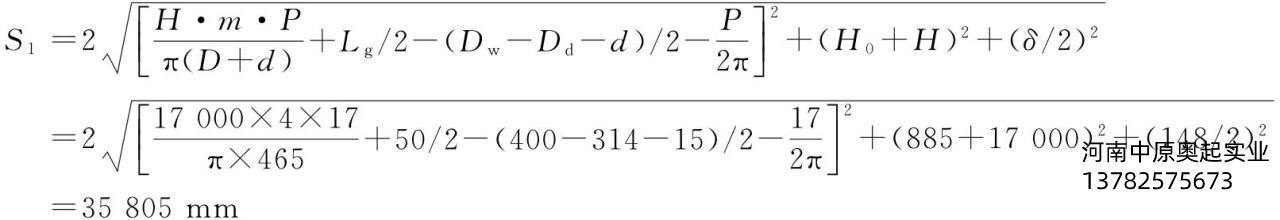b.钢丝绳在吊钩组动滑轮上的缠绕长度S2

S2=π(Dd+d)·m/2=3.14×(314+15)×4/2=2066mm

c.钢丝绳在小车横梁上定滑轮的缠绕长度S3

S3=π(Dg+d)·(m-2)/2=3.14×(314+15)×(4-2)/2=1033mm

d.钢丝绳在小车横梁上楔形套筒固定轴式超载限制器的总长度S4

S4≈3(Dg+d)/2=3(314+15)/2=494mm

e.钢丝绳在卷筒上的固定和安全圈数展开总长度S5

S5=10π(D+d)=10×3.14×(450+15)=14601mm

f.钢丝绳从动滑轮到小车横梁里的定滑轮总长度S6g.钢丝绳总长度

S=S1+S2+S3+S4+S5+S6

=35805+2066+1033+494+14601+106921

=160920mm=160.92m

加1～2m余量，一分为二，每根钢丝绳长度为82m。

设计某中欧式起重机小车，其设计参数为：最大额定起重量Q=100t;小车自重G1x=17t;工作级别A6;起升高度16m;起升速度V起=0.56～5.6m/min;小车运行速度V小=3～30m/min。

(1)100t低静空起重机小车横梁计算(图3-58)

根据起升机构的设计，布置定滑轮组的结构，得以下尺寸参数。

Q=1.0×106N;a=1250mm;I1=I4=1.1×109mm4;I2=I3=4.3×109mm4;S1=S4=2.8×106mm3;y2=y3=556mm;S2=S3=6.2×106mm3;t1=20mm;t2=20mm;t3=20mm;L=3600mm;m=8;主材采用Q235-B材料。强度符合要求。

根据小车横梁的结构设计截面尺寸(图3-58)，验算刚度。(2)100t大欧式低静空起重机小车端梁计算(图3-59)

根据小车机构布置，得以下小车端梁尺寸参数。

Q=1.0×106N;Gx=85000N;a=1400mm;b=3300mm;c=800mm;t1=t2=t3=24mm;t=330mm;h1=250mm;h=570mm;I1=I6=1.6×108mm4;I2=1.4×109mm4;I3=1.3×109mm4;I5=2.4×109mm4;y2=367mm;y3=260mm;y5=319mm;S1=S6=8.1×105mm3;S2=3.2×106mm3;S3=3.5×106mm3;S5=4.7×106mm3;主材采用Q345-B材料。小车端梁计算符合要求。

(3)小车运行机构设计计算(小车“三合一”减速器选型计算)

①小车摩擦阻力计算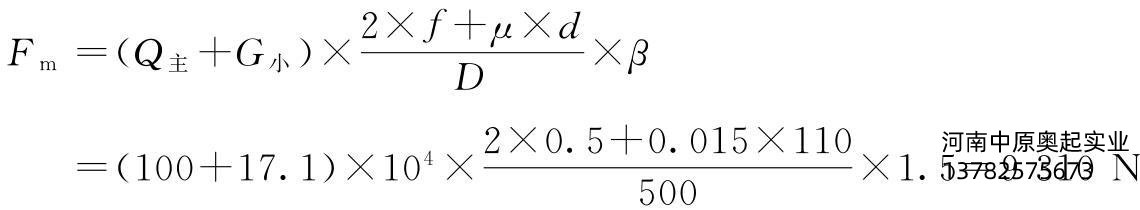②小车坡道阻力的计算

坡度角αsinα=0.001

坡道阻力Fp=(Q主+G小)×sinα=(1000000+171000)×0.001=1171N

③小车运行静阻力的计算

Fj=Fm+Fp=9310+1171=10481N

④小车运行静功率计算PN=kd·Pj=1.2×2.79=3.4kW(kd为经验系数)

⑤小车运行静阻力矩的计算

MP1=Fj×R=10481×0.25=2620N·m

式中　R——小车车轮的半径(m)，R=D/2。

小车满载直线运行产生的惯性力矩：加速度取0.05。MH=MP1+Mg1=2620+2240=4860N·m

小车为对边驱动型式，使用两个减速器驱动，1个齿轮箱应承载MH/2=2430N·m。

Na=V/3.14×D=19.1

⑥减速器选型

推荐选取国内恒星科技品牌“三合一”F系列减速器：功率4kW;fb=1.56;i=73.53;na=19;Ma=1950N·m。

Ma×fb=1950×1.56=3042N·m>MH/2=2430N·m;所选减速器符合要求。

⑦小车最大轮压计算

小车最大轮压是R1和R6的大值，R6=380kN>R1=230kN，所以，Pmax=R6=380kN为小车的最大轮压(主动轮压)，R1=230kN为被动轮压，R1/R6=230/380=0.61，被动轮、主动轮压比较理想，被动轮压比较接近主动轮压但小于主动轮压，在实践中一般不会出现持久打滑现象。

⑧小车车轮设计计算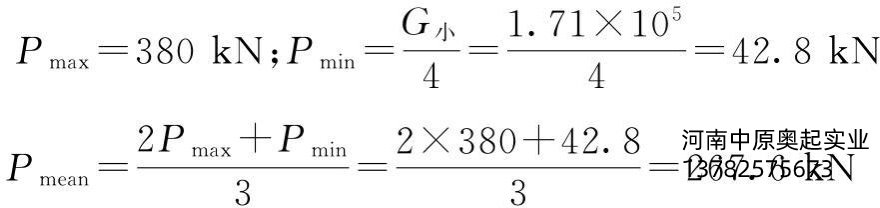小车轨道选方钢80mm×80mm，初选实心ф500mm直径车轮，车轮有效接触宽度l=80-10=70mm;

车轮材料选DG42CrMo，取σb=1080N/mm2，许用轮压比k=8.5

允许轮压PL=kDlC=8.5×500×70×1.06×0.9=284kN

疲劳强度校核：Pmean=267.8kN≤PL=284kN，满足要求。

静强度校核：380kN=Pmax≤1.9kDl=1.9×8.5×500×70=565kN，满足要求。

(4)起升机构的设计计算

①钢丝绳的选型计算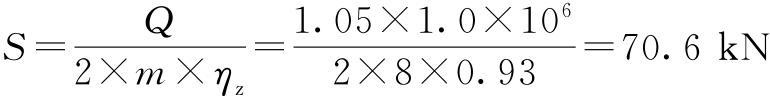所需钢丝绳最小破断拉力：S0=S×n=70.6×5.6=395.4kN

选择某国际品牌钢丝绳，查样本型号为：22-8×WS(26)+WSC-1960。

样本上钢丝绳最小破断拉力为433.7kN，所选钢丝绳符合要求。

②起升电机的选型计算

起升机构总传动效率η=0.85。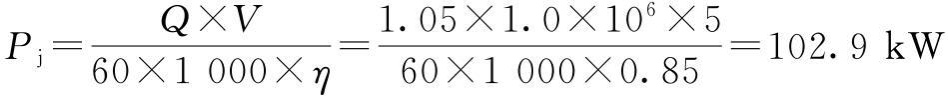选某国内品牌变频电机：YZP315M-6;110kW(S340%);985r/min;所选电机符合要求。

③起升减速器的选型计算

初步取卷筒名义直径尺寸：D=800mm

钢丝绳公称直径：d=22mm

卷筒计算直径：D0=d+D=22+800=822mm

起升动载系数：Φ2=1.12

减速器低速端所承受的扭矩为：

T=Φ2×S×D0=1.12×7.06×104×0.822=65kN·m

卷筒转速：减速器速比：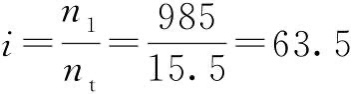确定齿轮箱的额定功率：P1N≥Pj·f1·f2·SF=102.9×1.1×1×1.25=141.5kW

最大启动扭矩：TA=1.8×Mn=1.8×1050.5=1890.9N·m(查电机样本得Mn)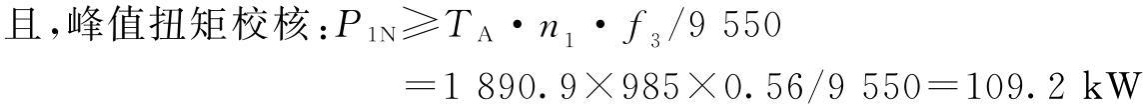选某国内品牌减速器：(额定功率146kW)i=63，减速器许可的短暂扭矩88kN·m。综上述计算结果可知，减速器的选择符合规范要求。

④制动器的选型计算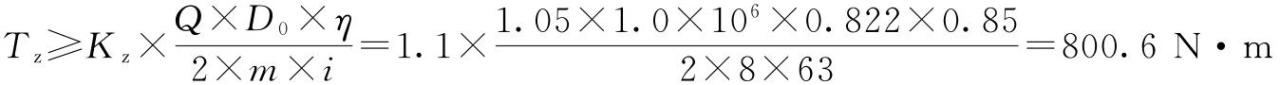起升选用双盘式制动器，YP1-500-Ed50/6-1000制动力矩1000N·m，符合设计要求。

⑤卷筒联轴器的选型计算

查工作级别系数K2=1.6(表3-6)卷筒支承轴承的效率，ηT=0.98。

卷筒钢丝绳总拉力∑S=(Q+Q0)/m×η=(1.05×1.0×106)/8×0.98=133.9kN

联接处所受实际转矩￼联接处的计算转矩

TK=T×K2×Φ6=56.2×1.6×1.06=95.3kN·m

联接处实际承受的径向载荷￼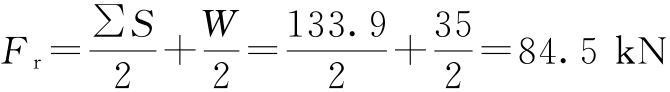选某国内品牌联轴器WJ10，Tmax=100kN·m，Fmax=120kN，符合设计要求

⑥卷筒设计计算

以下为卷筒光杆Lg长度部分计算。

在设计小车时得以下数据：b=20mm;c1=60mm;δ=224mm;H3=1345mm;Dd=600mm;Dg=600mm;Dw=860mm;Hf=600mm。取Hmin=2810mm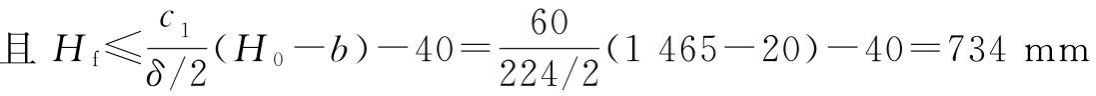H0=Hmin-H3=2810-1345=1465mm

Hf符合要求，钢丝绳在小车横梁底部不会发生干涉。

Dw-Dd-d≥Lg≥Dw-Dd-d-2H0·tan3.5°

860-600-22≥Lg≥860-600-22-2×1465×tan3.5°

即238≥Lg≥59mm，取：Lg=80mm

主卷筒选择：P=25mm，起升高度16m取卷筒长2900mm。小车轨距按3500mm设计。

⑦钢丝绳长度的计算

根据前面卷筒光杆部分计算，得H0=1465mm。

a.钢丝绳从卷筒绕出点到吊钩组最外缘动滑轮水平线上绳槽的绕入点的空间距离S1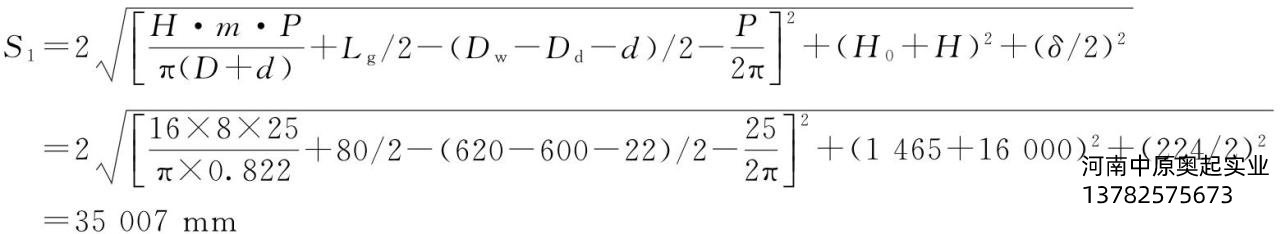b.钢丝绳在吊钩组动滑轮上的缠绕长度S2

S2=π(Dd+d)·m/2=π×(600+22)×8/2=7816mm

c.钢丝绳在小车横梁上定滑轮的缠绕长度S3

S3=π(Dg+d)·(m-2)/2=π×(600+22)×(8-2)/2=5862mm

d.钢丝绳在小车横梁上楔形套筒固定轴式超载限制器的总长度S4

S4≈3(Dg+d)/2=3(600+22)/2=933mm

e.钢丝绳在卷筒上的固定和安全圈数展开总长度S5

S5=10π(D+d)=10×π×(800+22)=25824mm

f.钢丝绳从动滑轮到小车横梁里的定滑轮总长度S6g.钢丝绳总长度S加1～2m余量，一分为二，每根钢丝绳长度为161m。

关键词：起重机